# 2.3.2. II ComplementBinary arithmetic uses different methods for calculating the forfeiture proceedings addition problem, and one of them is a SECOND COMPLEMENT (dual complement), for which the following rules apply:

```        1. Add subtrahend as "0" at the beginning how necessary
to have the same number of digits as minuend.
2. Subtrahend as I complement is determined so that the all
"0" the subtrahend into a "1" and "1" is converted to "0".
3. I complement subtrahend determined II complement
by being added to the amount 1.

II K = I K + 1

4. Minuend was counted II complement of the subtrahend.
In the sum the most significant bit is omitted.
The remaining difference is the required result.```

Omitted or ignored most significant bit is called the OVERFLOW and it is always "1". It should not be forgotten to leave at the end of the procedure.

Example II

Subtract decade numbers 15 and 5 by the method of II complement.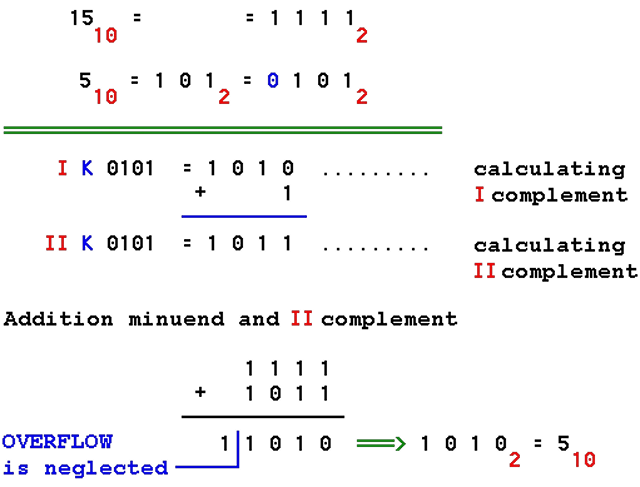Special operations with binary numbers in a computer are the SHIFT, and the ROTATION to the left or to the right. The principle of shear and rotation through a four-bit register (cache) is shown in the following scheme:By shift of contents of the register moves to the left or to the right and on the vacancies entered "0". Facilities that 'exit' from the registry, or dressing, are transferred to the other circuits or indication (flag) that indicates the changing conditions in a dedicated registry when it comes to the 'loss' of the first "1".

Rotation to the left or to the right amenities that come from the register automatically were entering on the other side.

Example III

Shift and rotation of binary number 110001B in eight-bit register in the term left and right side.1100012 = 001100012 ( = 49 decade )

a.) Shift to the left: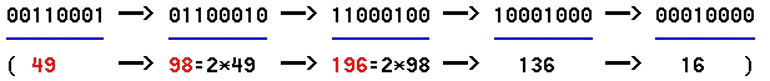Shift to the left results in twice the number of previous, until it reaches the overflow unit. So a fast multiply by two. In case of overflow units, overflow signals the change of content in a dedicated register.

b.) Shift to the right: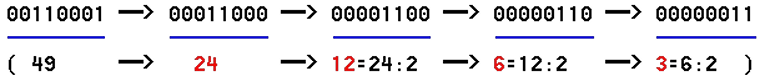Shift to the right results in half the number of previous, until it reaches the overflow unit. So a fast division by two. Overflow "1" is also the remainder of division.

c.) Rotation to the left: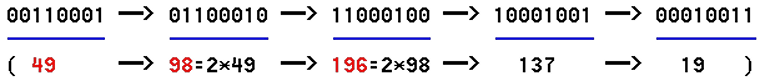Rotation to the left has the same effect as a shift to the left to overflow unit.

d.) Rotation to the right: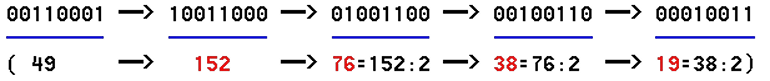Rotation to the right has the same effect as a shift to the right to overflow unit.

The computer are enabled at shift relatively complex issues, but it is important to know when to lead bit and when it will end is not lost but is stored in one of the computer's temporary memory.SUMMARY:

How does all calculations in the computer translate in summation that the adjusted system of marking numbers in a way that represents the MSB-bit prefix ("1" for negative and "0" for positive number), and all negative numbers will come up with the remaining 's displayed as II complement the natural binary sequence.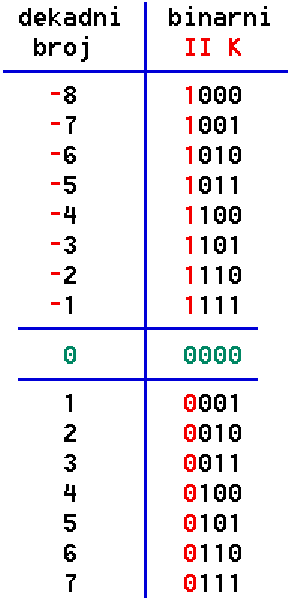In this way defines the zero ( 0000 ). According to the above table shows that the range of negative numbers are slightly higher compared to the positive. MSB bit over arithmetical operations are kept in a special container (register) of your CPU so that it knew how to act with a binary number to a record in the tank. In the structure of the processor (Central Processing Unit - CPU) contains more registers intended for calculations. About all operations in the logical structure of the processor takes care of the arithmetic-logic unit called the ALU (Arithmetic and Logic Unit), the main gate to the CPU.

So, register capacity of 8 bits can hold whole numbers from 01111111 to 10000000 (+127 to -128) and if it does not exceed the specified limits can be inside them perform integer math operations (integer). If there are two 8-bit register that they are unified in one 'bigger' can be used range from 0111111111111111 to 1000000000000000 (+32767 to -32768) within which to perform integer math operations (long integer). But what about larger numbers or real numbers? You can not use more registers than physically offered the aligned structure of the processor. The decade system of real numbers are displayed so that a point dividing the entire portion of the fractional part. 2.54, 25.4 and 0.0254 are examples of real decade numbers. Such a mode is called a record with a fixed point. As is impractical for very large or very small numbers, but rather uses exponential numbers or track, with a record with a moving point, which contains a mechanism sign (s), base (b), an exponent (e) and precision (p).

Number 3.1415927410125732421875 can be represented as e=0; +3.141592 or e=-2; +314.1592; or e=+4; +0.000314 (prefix s=+, precision p=8, base b=10). The example is presented in decade system of numbers that are easier to accept the notion of a decimal point that 'moves' as the exponent. Precision is the 'cut' part of decimals displayed number. The same logic applies in the binary number system. The sign determines the MSB as shown in the previous table. So, one part of the tank will contain information on the sign, the exponent and the precision and the rest will be given to the number of digits of precision. If you take a number of places for precision, there is less for the size of the exponent. So with greater precision falls available for computing range without overflow or excess capacity (overflow). Division by zero is unacceptable operations. For these fairly demanding special care procedures logical structure of a process called math coprocessor, or more precisely, units for computing with floating-point (Floating Point Unit - FPU). The first versions of the processor are designed with two physically separate chip, CPU and FPU. FPU is to buy more if needed and put into the already prepared for him stand. Certain types of software can not work without FPU (such as AutoCAD). In the early era of microprocessor FPU work, if needed, 'starred' is a special utility programs which carried out the CPU, which is then the entire computer system seemed very slow. Today's microprocessors have an ALU and FPU unified into a single chip as illustrated by Figure 3.5.3. Stated regarding the number range and precision beautifully illustrated picture in the Chapter 6.2.6 of QBASIC's.

In short, the computer processor performs a variety of mathematical operations in a binary system of numbers by the logical sequence according to a set of instructions that it be delivered to you by the internal logical structure of the processor, i.e. its logic circuits.Citing of this page: Radic, Drago. " IT - Informatics Alphabet " Split-Croatia. {Date of access}. . Copyright © by Drago Radic. All rights reserved. | Disclaimer